Technical Article

# Digital Image Processing: Point Operations to Adjust Brightness and Contrast

October 31, 2019 by Dr. Steve Arar

## In this article, we’ll first look at the basics of representing a digital image. Then, we’ll discuss some simple operations that allow us to adjust the brightness and contrast of an image. Finally, we’ll briefly look at the circuit implementation of these operations.

Digital image processing involves developing algorithms that can be used to enhance a given image or extract some useful information from it.

In this article, we’ll first look at the basics of representing a digital image. Then, we’ll discuss some simple operations that allow us to adjust the brightness and contrast of an image.

Finally, we’ll briefly look at the circuit implementation of these operations.

### Digital Image Structure

A digital image can be described by a two-dimensional array of small elements called pixels (a contraction of the phrase picture elements). For example, many commercially available imaging devices produce images of approximately 512 x 512 pixels. The value of each pixel relates to the energy that our imaging device receives from the source of radiation. It’s important to note that the radiation doesn’t have to be from the visible light band. In fact, the different bands of the electromagnetic spectrum can be used to glean the fullest possible amount of information from the world around us. One common method to represent a digital image is to store each pixel value as an unsigned N-bit number. For example, a grayscale image may use 8 bits to represent the value of a pixel. In this case, the value of each pixel can vary from 0 that represents black to 255 that relates to white. The intermediate values correspond to the different shades of gray. Figure 1 shows a portion of a grayscale image along with its corresponding pixel values.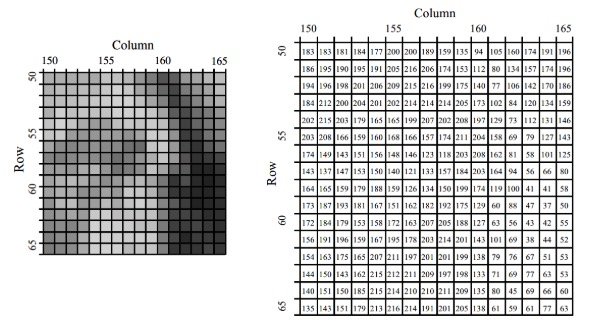##### Figure 1. Image courtesy of The Scientist and Engineer's Guide to Digital Signal Processing.

Based on the above discussion, we can represent a grayscale image as a two-dimensional function I[x, y] where the arguments x and y are the plane coordinates that specify a particular pixel of the image. The value of the function determines the intensity or gray level of the image at that point.

### Point Operations on a Single Image

Point operations modify a given pixel based on its value and possibly its position. These operations can be represented by a mapping or transfer function. For example, assume that the gray levels of an image are represented by I[x, y]. We can use an arbitrary function, fxy, to map each of these gray levels to a new value Q[x, y]. This can be represented by the following equation:

Q[x, y] = fxy (I[x, y])

The indices of the mapping function denote the dependency of the function on the pixel position. If the function doesn’t depend on the pixel position and depends only on the pixel value, we can remove the indices and obtain

Q[x, y] = f (I[x, y])

A mapping function can be described graphically. An example function is shown in Figure 2 below.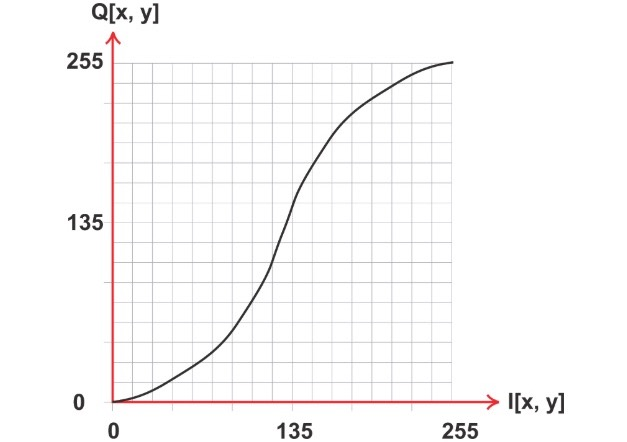##### Figure 2

Another example is shown in Figure 3. The output of this transfer function is the same as its input value (it doesn’t change the image). This mapping doesn’t seem to be of much practical interest, but can help us better understand the transfer functions that we’ll discuss next.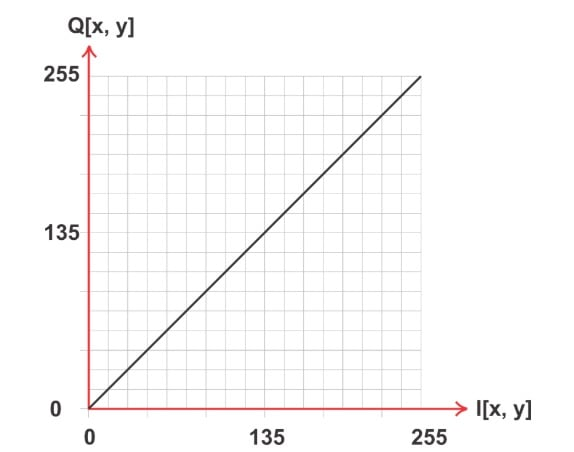##### Figure 3

We can use point operations to adjust the contrast and brightness of an image, perform thresholding, image averaging, and more. Below, we’ll discuss the transfer functions for adjusting the brightness and contrast of an image.

To adjust the brightness of an image, we change the value of all pixels by a constant. Adding a positive constant to all of the image pixel values makes the image brighter. Similarly, we can subtract a positive constant from all of the pixel values to make the image darker. Figure 4 shows an example transfer function that can be used to increase the brightness of an image. This transfer function increases all the pixel values between 0 and P1 by a constant value b. For pixel values greater than P1, the addition result will be greater than the maximum possible value that can be represented by an eight-bit number. Hence, these values are saturated at the maximum value (255 in this example).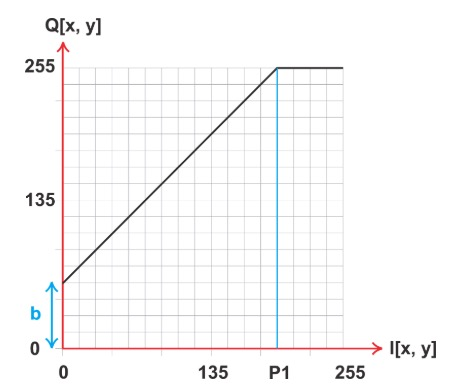##### Figure 4

Figure 5 illustrates how adding a positive constant to the Lena image can make it brighter. For the brighter version, the constant 70 is added to the pixel values.##### Figure 5

An example transfer function that reduces the brightness of an image is shown in Figure 6.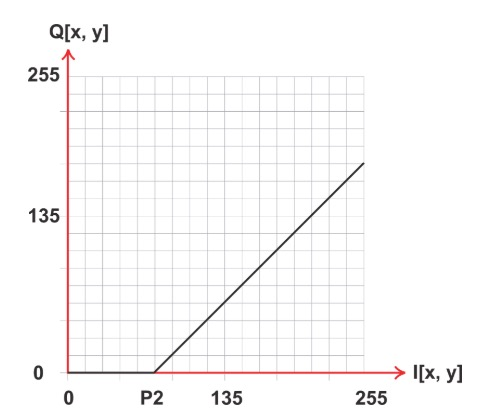##### Figure 6

Figure 7 shows the effect of adding the negative constant -70 to the Lena image.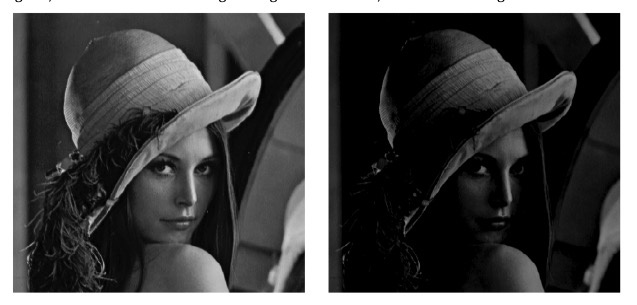##### Figure 7

While brightness refers to the overall lightness or darkness of an image, contrast refers to the brightness difference between different objects or regions of the image. To adjust the contrast, we can modify the slope of the transfer function. A transfer function with a slope greater than one will increase contrast, while a slope less than one will decrease it. Figure 8 shows an example transfer function with a slope greater than one.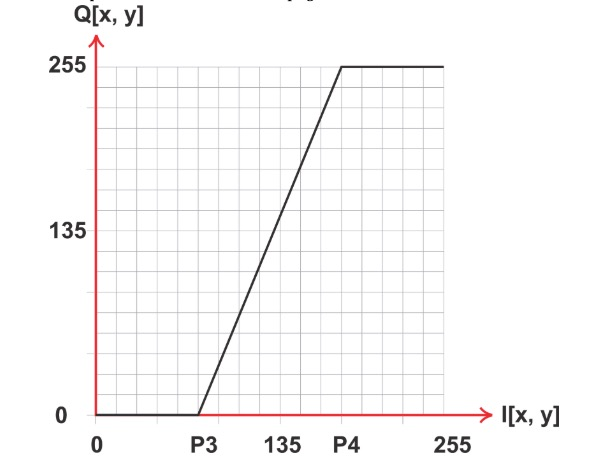##### Figure 8

In this example, the pixel values that are near but less than P4 are mapped to values just below 255 (they become brighter). The pixel values close to but greater than P3 are mapped to values just above 0 (they become darker). Hence, the above transfer function increases the contrast of the pixels whose value is between P3 and P4. Note that all pixel values below P3 are mapped to zero and the pixel values above P4 are mapped to 255. Since all the pixel values outside the range from P3 to P4 are represented by either black or white, we have lost the information in these regions. Therefore, a given contrast enhancement function may improve the viewability of certain regions of the image yet degrade it at pixel values near 0 and 255. By appropriately choosing the value of P3 and P4 for a given image, we might be able to more easily observe a certain feature of the image.

### Conclusion

In this article, we first looked at the basics of representing a digital image. Then, we discussed some simple point operations that allow us to adjust the brightness and contrast of an image.